# 3D Visibility made Visibly Simple

#### Transcription of a video of the 13rd ACM Symposium on Computational Geometry, Nice, France, June 97In this work we are interested in visibility queries such as the computation of vertex-to-polygon view or limits of umbra and penumbra caused by a polygonal light source.

## The Visibility Skeleton

Our work is based on the characterisation of the topological changes of visibility.

For example, here, the polygon seen behind the highlighted vertex changes as we move. This change occurs when the vertex and the edge are aligned from the viewpoint.The locus of such changes is defined by the set of lines going through the vertex and the edge. This is a one dimensional set of lines, and can be parameterized for example by the intercept on the edge. We call it a critical line swath. We refer to it as an EV line swath, indicating the edge-vertex pair.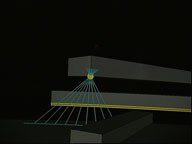Now consider the extremities of such a 1D set. Here we have two vertices aligned. There is only one line through two points. There are zero degrees of freedom It is a zero dimensional set.

Upper left we show the adjacencies between the critical line swath and the extremal stabbing lines.

At the other extremity of the swath, we have a vertex aligned with two edges.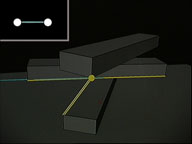These are two examples of extremal stabbing lines, a VV (vertex-vertex) and a VEE (vertex-edge-edge).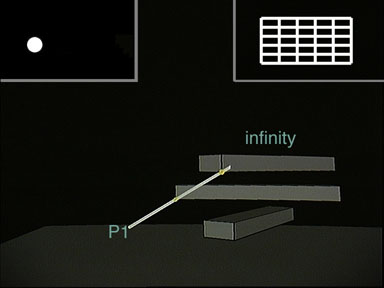Consider this extremal stabbing line. There is another critical line swath emanating from it involving the same edge and vertex, but with a different polygon behind.

Yet another EV swath.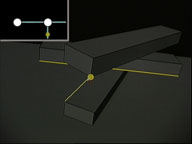Here we have 3 edges aligned, resulting in a topological change in visibility.

Yet another triple-edge line swath.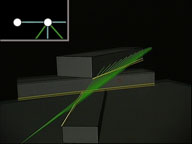An extremal stabbing line can be seen as the node of a graph, and the critical line swaths are its arcs.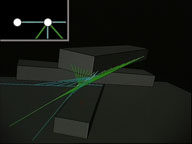For each kind of node, we define the catalogue of adjacent arcs. For example, VV nodes have an EV arc generated by each edge adjacent to one of the 2 generator vertices.Each node or arc is characterised by the polygons at its extremities. This polygon can be null if the extremity is at infinity.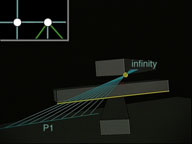The arcs of our graph are stored in a two-dimensional array of binary search trees indexed by the polygons at the extremities. This array of trees represented upper right allows for efficient queries.We call the graph of critical line swaths and extremal stabbing lines together with this array the Visibility Skeleton.

## construction algorithm

To construct the skeleton we enumerate all the nodes and attach them the arcs using the array.

We enumerate the generator stabbing line with nested loops on the vertices and edges of the scene.

Each potential extremal stabbing line is then tested for occlusion by ray-casting.

If no object lies between the generators of the extremal stabbing line, a node is created. The ray-casting operation also returns the two objects at the extremities.

For each adjacent arc, we check if it already exists in the [list/tree] of the array indexed by its extremities. If not, the arc is created.

Notice here that the polygon P2 at the extremity of the arc is different from that of the node.

Here The arc had already been created and thus is attached to the node.

And so on...

## Queries

#### exact point-to polygon view

If we want to know the part of a polygon F seen from a vertex v, we search for the arcs with generator v which have an extremity lying on F.

#### face-to-face discontinuity meshing

We now consider one polygon as a light source, and another one as a receiver.

We compute the limits of umbra and penumbra by considering the arcs whose extremities are respectively the source and the receiver and also those which have a generator on the source and an extremity on the receiver.

We now demonstrate these queries on a more complex scene.